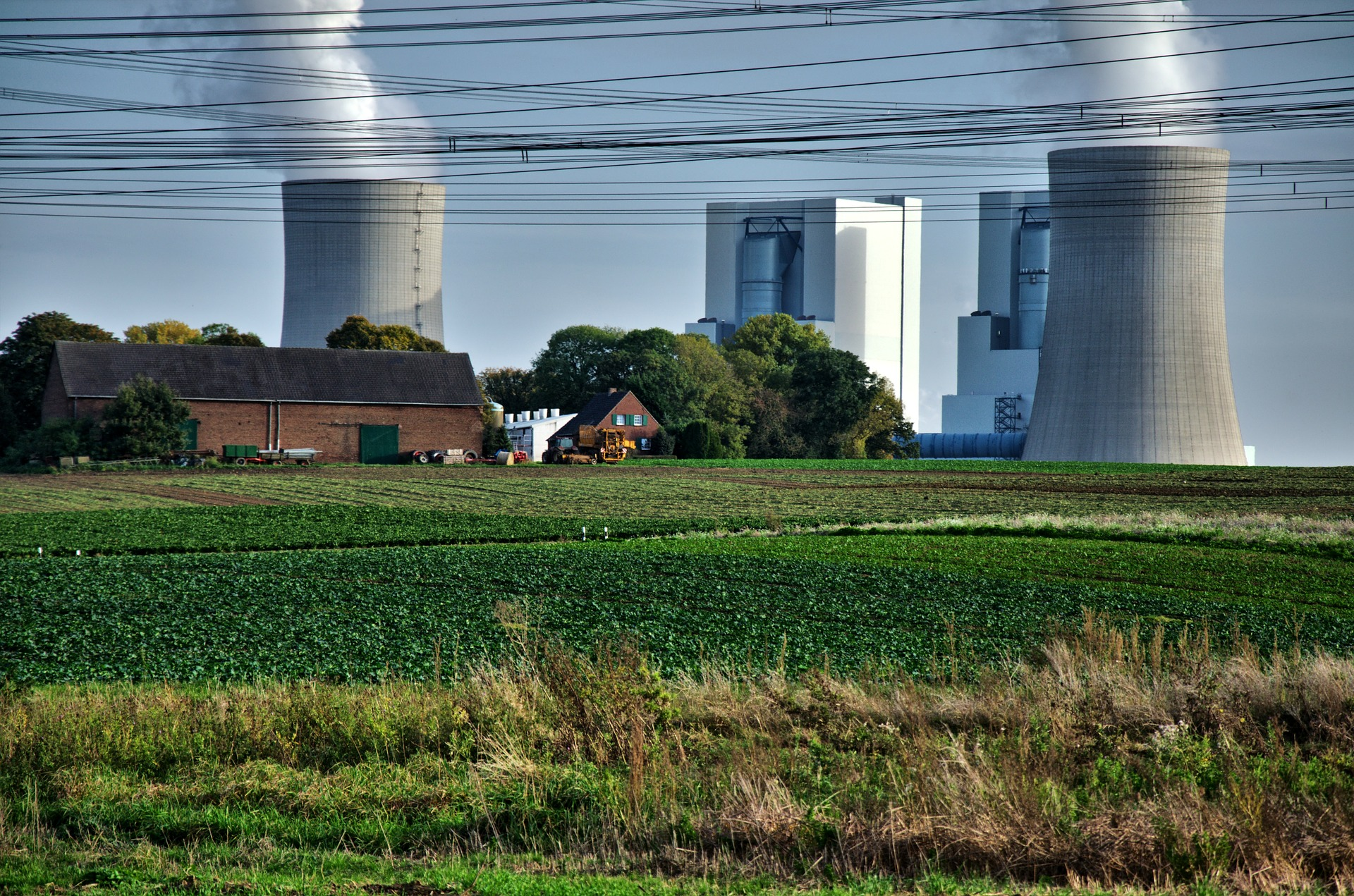# Cooling Tower Makeup Water Calculation with Example

It’s been a while since the last time I write an article about utility system. In this post I want to share you how to calculate cooling tower makeup water.

Cooling tower must be made up because the water level decrease by the time during operation. There are aspect that contribute to calculation of cooling tower makeup water. Those are:

• Evaporation loss
• Drift loss
• Blow down

Total cooling water makeup water requirement = evaporation loss + drift loss + blow down.

Let’s take a look one by one how to calculate each component.

### Evaporation Loss

Evaporation loss is loss of water due to evaporation. It is calculated by this equation:

Evaporation loss = 0.00085 Wc (T1-T2)

Where:

T1 – T2 = inlet water temperature minus outlet water temperature (oF)

0.00085 is evaporation constant (rule of thumb). The actual evaporation rate is a function of season and climate.

### Drift Loss

Drift is entrained water in the tower discharge vapor. Drift loss in cooling tower is a function of drift eliminator design.

Drift loss = 0.02% water supply [for new development in eliminator design]

### Blowdown

Blowdown is a portion of circulating water that is discharged in order to lower solids concentration due to evaporative cooling. The requirement of blowdown is related with cycle of concentration (COC). COC is best described as ratio of chloride content in circulation water and in makeup water.

Blowdown = [ Evaporative loss – (COC – 1) x Drift loss] /(COC – 1)

Cycle of concentration is normally 3-4 cycles. When cycle of concentration below 3 cycles, quantity of water to be discharged will be large.

## EXAMPLE

Let say we have cooling tower with the following data:

Inlet water flow rate = 10 000 gpm

Inlet water temperature = 100 oF

Outlet water temperature = 85 oF

Drift loss = 0.02%

Cycles of concentration = 5

Recall again, cooling tower makeup water = evaporation loss + drift loss + blowdown. Let’s calculate each component.

• Evaporation loss = 0.00085 x 10 000 x (100-85) = 127.5 gpm
• Drift loss = 0.02% x 10 000 = 2 gpm
• Blowdown = [127.5 – (5-1) x 2]/(5-1) = 29.87 gpm
• Cooling tower makeup water = 127.5 + 2 +29.87 = 159.37 gpm

Reference:

Perry’s Chemical Engineers’ Handbook

## 14 thoughts to “Cooling Tower Makeup Water Calculation with Example”

1.abdulmannan says:

is it same for natural & mechanical draft cooling tower ?

1.Rifka Aisyah says:

Yes, it is the same.

1.Ava says:

How can we calculate the Per ton per hour make up water quantity ?

1.Rifka Aisyah says:

Hi,

m3/hr water quantity is the same as ton/hr water.

2.Alex Arnold says:

I’m impressed with this article. I’m a mechanical engineer doing HVAC everyday doesn’t come up every day. Matches the spreadsheet I created a couple of years ago.

3.Mahmoud Siaj says:

From experience there is difference between makeup & blowdown calculations based on average vs. peak. To clarify:

when calculating the blowdown there is peak and average consumption? which one we need to considered

4.Patrick okila says:

I like this calculation,much pleased and understood,

5.sara says:

i need to know the GPM calculated is per what ??
159.37 gpm will be make up water per day or hour or what ?

Thanks alot

1.Rifka Aisyah says:

Hi Sara,

Gpm is gallon per minute

1.Fatimah says:

157gpm will be approx. 95,000 gpd (assume at 10hrs operation) is a big number for make volume don’t you think so ? equivalent to 300 units of residential units water consumption. is this efficient ?

1.B says:

Efficiency is by Cycles of Concentration, depending on silica, water chemistry and % load

6.Nicolas says:

Hello, I need to know the Gpm for a 20 story office building, is there a way to have an aproximate size?

Floor is 1000 square meters and with 3.3 meters high

7.Ryan says:

Hello, I would like to know where is the make up water calculation formulas come from? CTI?

1.Rifka Aisyah says:

The reference is from Perry’s Chemical Engineers Handbook